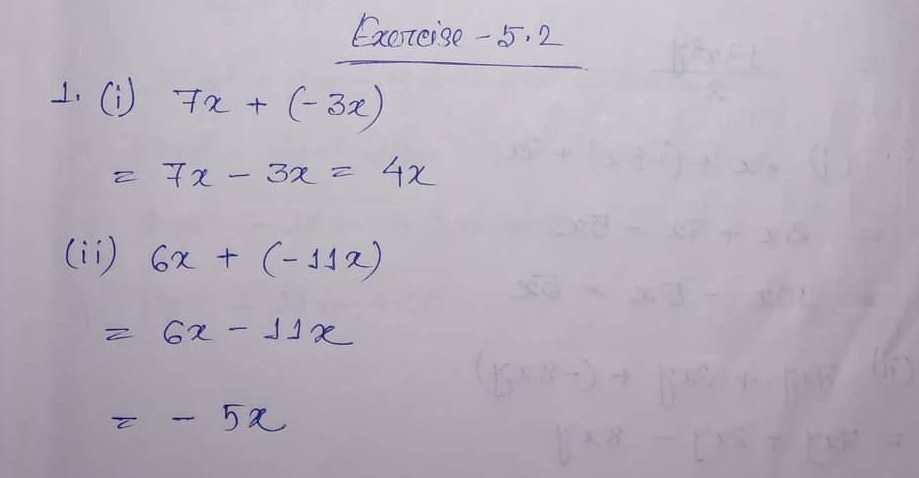# ML Aggarwal CBSE Solutions Class 7 Math Fifth Chapter Algebraic Expressions Exercise 5.2

### ML Aggarwal CBSE Solutions Class 7 Math 5th Chapter Algebraic Expressions Exercise 5.2

Exercise 5.2 Solution

(1) 7x, -3x

(ii) 6x, -11x

(iii) -9x2

(iv) 3ab2, -5ab2

(v) 1/2 pq, -1/3 pq

(vi) 5x3y, -2/3 x3y

(i) 3x, -5x, 7x

(ii) 7xy, 2xy, -8xy

(iii) -2abc, 3abc, abc

(iv) 3mn, -5mn,8mn, -4mn

(v) 2x3, 4x3, -4x3, -5x3

(3) Simplify the following combining like terms:

(i) 21b – 32 + 7b – 20b

(ii) 12m2 – 9m + 5m – 4m2 – 7m + 10

(iii) –z2 + 13z2 – 5z + 7z3 – 15z

(iv) 5x2y – 5x2 + 3yx2 – 3y2 + x2 – y2 + 8xy2 – 3y2

(v) p – (p – q) – (q – p) – q

(vi) 3a – 2b – ab – (a – b + ab) + 3ab + b – a

(vii) (3y2 + 5y – 4) – (8y – y2 – 4)

(4) Find the sum of the following algebraic expressions:

(i) 5xy, -7xy, 7x2

(ii) 4x2y, -3xy2, -5xy2, 5x2y

(iii) -7mn + 5, 12mn + 2, 8mn – 8, -2mn – 3

(iv) a + b – 3, b – a + 3, a – b + 3

(v) 14x + 10y – 12xy – 13, 18 – 7x – 10y + 8 xy, 4xy

(vi) 5m – 7n, 3n – 4m + 2, 2m – 3mn – 5

(vii) 3x3 – 5x2 + 2x + 1, 3x – 2x2 – x3

(viii) 7a2 – 5a + 2, 3a2 – 7, 2a + 9, 1 + 2a – 5a2

(5) Simplify the following:

(i) 2x2 + 3y2 – 5xy + 5x2 – y2 + 6xy – 3x2

(ii) 3xy2 – 5x2y + 7xy – 8xy2 – 4xy + 6x2y

(iii) 5x4 – 7x2 + 8x – 1 + 3x3 – 9x2 + 7 – 3x4 + 11x -2 + 8x2

(6) Subtract:

(i) -5y2 from y2

(ii) – 7xy from -2xy

(iii) a(b – 5) from b(5 – a)

(iv) –m2 + 5mn from 4m2 – 3mn + 8

(v) 5a2 – 7ab + 5b2 from 3ab – 2a2 – 2b2

(vi) 4pq – 5q2 – 3p2 from 5p2 + 3q2 – pq

(vii) 7xy + 5x2 – 7y2 + 3 from 7x2 – 8xy + 3y2 – 5

(viii) 2x4 – 7x2 + 5x + 3 from x4 – 3x3 – 2x2 + 3

(7) Subtract p – 2q + r from the sum of 10p – r and 5p + 2q.

(8) From the sum of 4 + 3x and 5 – 4x + 2x2, subtract the sum of 3x2 – 5x and –x2 + 2x + 5

Solution Number 1, 2, 3, 4, 5, 6, 7 & 8:(9) What should be added to x2 – y2 + 2xy to obtain x2 + y2 + 5xy?

(10) What should be subtracted from -7mn + 2m2 + 3n2 to get m2 + 2mn + n2?

(11) How much is y4 – 12y2 + y + 14 greater than 17y3 + 34y2 – 51y + 68

(12) How much does 93p2 – 55p + 4 exceeds 13p3 – 5p2 + 17p – 90?

(13) What should be taken away from 3x2 – 4y2 + 5xy + 20 to obtain – x2 – y2 + 6xy + 20?

Solution Number 9, 10, 11, 12 & 13:(14) From the sum of 2y2 + 3yz, y2 – yz – z2 and yz + 2z2, subtract the of 3y2 – z2 and –y2 + yz + z2

Solution Number 14:Updated: January 7, 2020 — 11:05 am